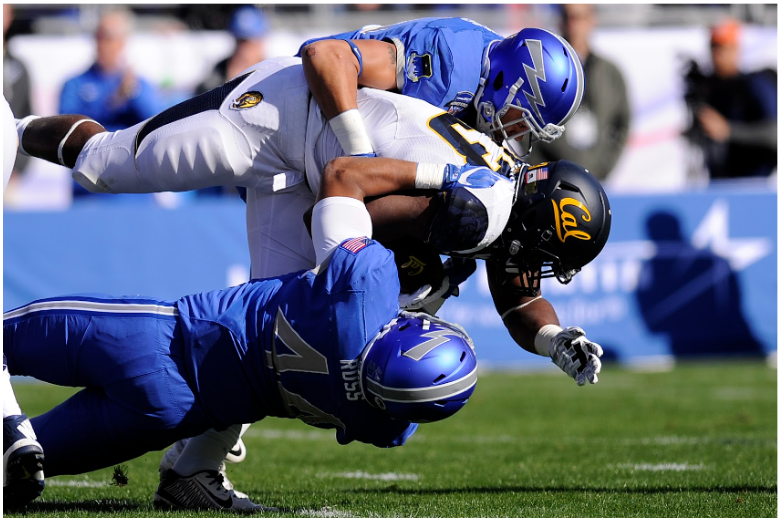# Applications of Calculus: Calculating the Horsepower of an Offensive Lineman

It comes out to roughly a fortieth of that of a truck.

This post is part of a series.Hearing the word “horsepower,” many people think of car engines. Horsepower measures the rate of work, or energy expenditure, so it is commonly used as a performance metric to compare engines.

However, horsepower is not a familiar metric like height or weight. We know the heights and weights of everyday objects, so it is easy for us to know that 1000 pounds is very heavy to lift, and a person who is 4 feet tall is considered short. But when we say that a pickup truck has 300-400 horsepower, what can we compare it to?

Do you know the horsepower of a human, say, an NFL offensive lineman?

Let’s find out.

An offensive lineman’s power can be calculated as the product of his force and maximum velocity:

\begin{align*} P = Fv_{max} \end{align*}

And force is given by the product of his mass and his acceleration:

\begin{align*} F = ma \end{align*}

Mass is easy to find: a typical lineman weighs about $320$ pounds, or $145$ kilograms. Acceleration and maximum velocity are more difficult to find, but using calculus, we can find them from a lineman’s $40$-yard dash time.

Say the lineman runs a $40$-yard dash in $5.3$ seconds, reaching top speed $2$ seconds after starting. $40$ yards is $36.6$ meters, so we have

\begin{align*} 36.6 = (\mbox{distance while accelerating}) + (\mbox{distance at top speed}) \end{align*}

First, we need to use calculus to solve for the distance while accelerating. Since acceleration is the derivative of velocity, velocity is the integral of acceleration:

\begin{align*} a = \frac{dv}{dt} \Rightarrow v(t) = \int a dt \end{align*}

Therefore, the velocity is given by

\begin{align*} v(t) = at + C. \end{align*}

where $C$ is a constant. But since the velocity at time $t = 0$ is $0,$ we have

\begin{align*} 0 &= v(t=0) \\[5pt] &= a(0) + C \\[5pt] &= C \end{align*}

and hence the velocity is given by

\begin{align*} v(t) = at \end{align*}

Likewise, since velocity is the derivative of distance, distance is the integral of velocity:

\begin{align*} v(t) = \frac{dx}{dt} \Rightarrow x(t) = \int v(t) dt \end{align*}

Therefore, the distance is given by

\begin{align*} x(t) &= \int v(t) dt \\[5pt] &= \int at dt \\[5pt] &= \frac{a}{2}t^2 + C \end{align*}

where $C$ is again a constant.

Again, since the distance at time $t = 0$ is $0,$ we have

\begin{align*} 0 &= x(t=0) \\[5pt] &= \frac{a}{2}(0)^2 + C \\[5pt] &= C \end{align*}

and hence the distance is given by

\begin{align*} x(t) = \frac{a}{2}t^2 \end{align*}

Since the lineman stops accelerating at $2$ seconds, the distance covered during this period of acceleration is given by

\begin{align*} x(2) &= \frac{a}{2}(2)^2 \\[5pt] &= 2a \end{align*}

Now, we need to find the distance at top speed. The lineman is at top speed for $5.3 - 2 = 3.3$ seconds, and is traveling at a top speed of

\begin{align*} v_{max} &= v(2) \\[5pt] &= 2a \end{align*}

so the distance covered is given by

\begin{align*} \mbox{distance at top speed} &= v_{max}(3.3) \\[5pt] &= (2a)(3.3) \\[5pt] &= 6.6a \end{align*}

Substituting back into our original equation, we have

\begin{align*} 36.6 &= (\mbox{distance while accelerating}) + (\mbox{distance at top speed}) \\[5pt] 36.6 &= 2a + 6.6a \\[5pt] 36.6 &= 8.6a \\[5pt] 4.6 &= a \end{align*}

The lineman accelerates at $4.6$ $m/s^2.$

Also, solving for maximum velocity:

\begin{align*} v_{max} &= 2a \\[5pt] &= 2(4.6) \\[5pt] &= 9.2 \end{align*}

The lineman’s maximum speed is at $9.2$ $m/s.$

Plugging in to find the lineman’s power, we have

\begin{align*} P &= Fv_{max} \\[5pt] &= mav_{max} \\[5pt] &= (145)(4.6)(9.2) \\[5pt] &= 6136 \end{align*}

This measurement is in watts. Converting to horsepower, we divide by the conversion factor $745.7 W = 1hp,$ reaching $8.2$ horsepower, roughly a fortieth of that of a truck.

This post is part of a series.

Tags: